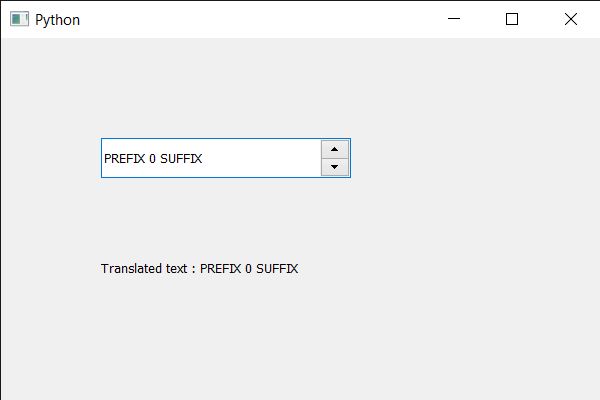# PyQt5 QSpinBox – Getting translated version of text

• Last Updated : 28 May, 2020

In this article we will see how we can get the translated version of the text. Translated version of text is based on the disambiguation string and value of n for strings containing plurals. If no appropriate translated string is available translated text will be a UTF-8 string i.e a Unicode codec and can represent all characters in a Unicode string like QString. However, invalid sequences are possible with UTF-8 and, if any such are found, they will be replaced with one or more “replacement characters”, or suppressed.

In order to do this we use `tr` method with the spin box object.

Syntax : spin_box.tr(text, d_text, n)

Argument : It takes 3 arguments first one is the text and other two are not compulsory first is disambiguation string and other is the integer that refer to the plurals number

Return : It returns string

Below is the implementation

 `# importing libraries``from` `PyQt5.QtWidgets ``import` `*` `from` `PyQt5 ``import` `QtCore, QtGui``from` `PyQt5.QtGui ``import` `*` `from` `PyQt5.QtCore ``import` `*` `import` `sys`` ` ` ` `class` `Window(QMainWindow):`` ` `    ``def` `__init__(``self``):``        ``super``().__init__()`` ` `        ``# setting title``        ``self``.setWindowTitle(``"Python "``)`` ` `        ``# setting geometry``        ``self``.setGeometry(``100``, ``100``, ``600``, ``400``)`` ` `        ``# calling method``        ``self``.UiComponents()`` ` `        ``# showing all the widgets``        ``self``.show()`` ` `        ``# method for widgets``    ``def` `UiComponents(``self``):``        ``# creating spin box``        ``self``.spin ``=` `QSpinBox(``self``)`` ` `        ``# setting geometry to spin box``        ``self``.spin.setGeometry(``100``, ``100``, ``250``, ``40``)`` ` `        ``# setting range to the spin box``        ``self``.spin.setRange(``0``, ``999999``)`` ` `        ``# setting prefix to spin``        ``self``.spin.setPrefix(``"PREFIX "``)`` ` `        ``# setting suffix to spin``        ``self``.spin.setSuffix(``" SUFFIX"``)`` ` `        ``# setting status tip to the spin box``        ``self``.spin.setStatusTip(``"Small Value"``)`` ` `        ``# creating a label``        ``self``.label ``=` `QLabel(``self``)`` ` `        ``# making label multi line``        ``self``.label.setWordWrap(``True``)`` ` `        ``# setting label geometry``        ``self``.label.setGeometry(``100``, ``200``, ``250``, ``60``)`` ` `        ``# translating the text``        ``value ``=` `self``.spin.tr(``self``.spin.text())`` ` `        ``# setting text to the label``        ``self``.label.setText(``"Translated text : "` `+` `str``(value))`` ` ` ` `# create pyqt5 app``App ``=` `QApplication(sys.argv)`` ` `# create the instance of our Window``window ``=` `Window()`` ` `# start the app``sys.exit(App.``exec``())`

Output :My Personal Notes arrow_drop_up